# PLY Format Reversing - Part 2 MoW:Vietnam

01-08-2017 - 5 minutes, 45 seconds -

DISCLAIMER: The information provided here is for educational purposes only.

# Introduction

I am a huge fan of the Men of War series, and with the release of Assault Squad 2 I decided to have a little fun and do some reverse engineering of the game's 3d file format. If you missed the first part of this series, check it out here. In this entry, I tackle some of the 3D formats found in MoW:Vietnam.

# Reconnaissance

First, I spawned a Vietnamese smg squad. They carry the venerable AK 47. The files for this weapon can be found in C:\Steam\steamapps\common\Men of War - Vietnam\resource\entity\e3.pak\inventory-weapon\ak47. The models associated with the AK47 are ak-47.ply, ak-47_lod1.ply, and ak-47_lod2.ply. Then I took a frame capture with the Intel Graphics Analyzer. Since I have set the game video settings to high, the high poly version of the model is used.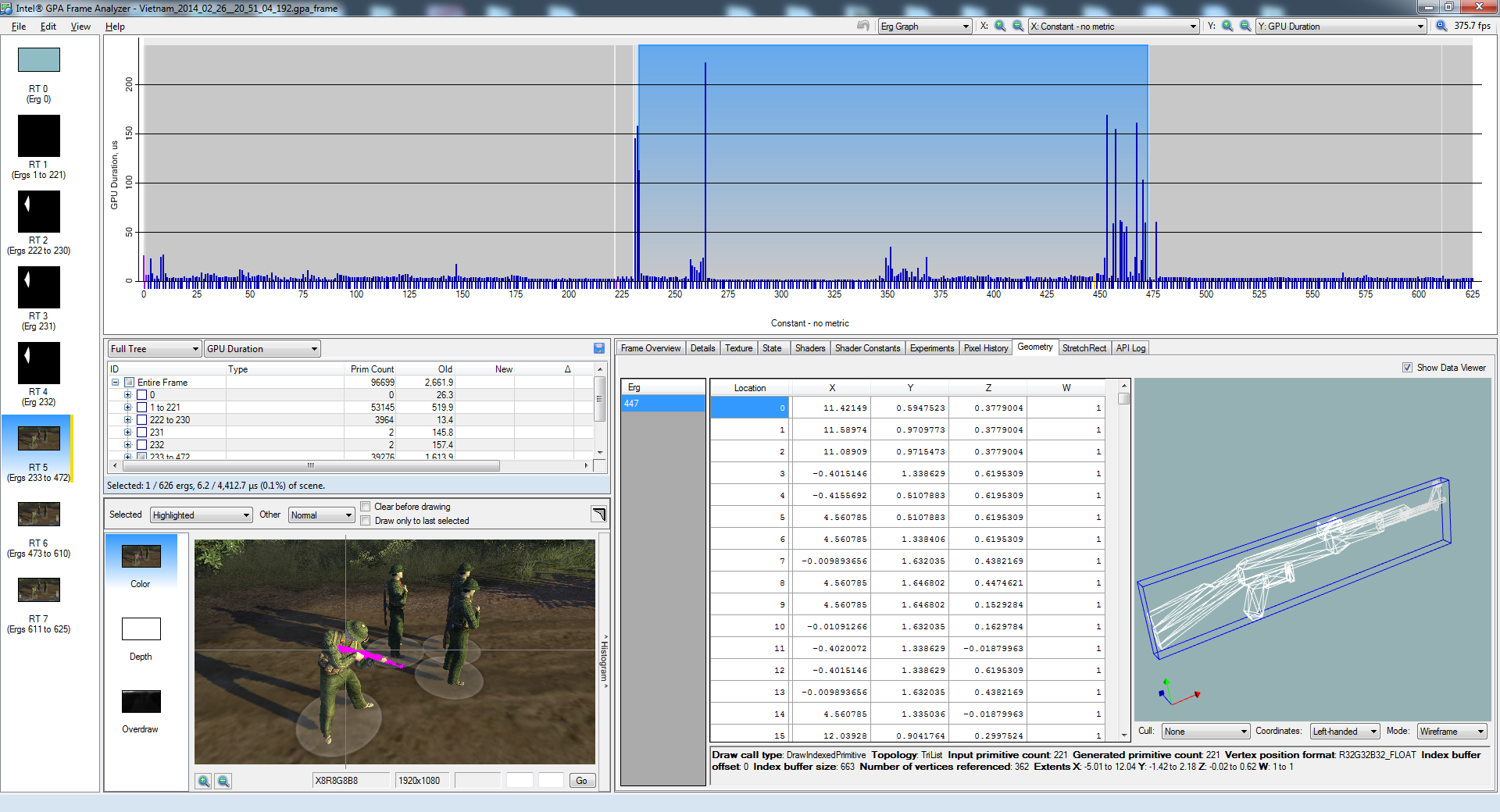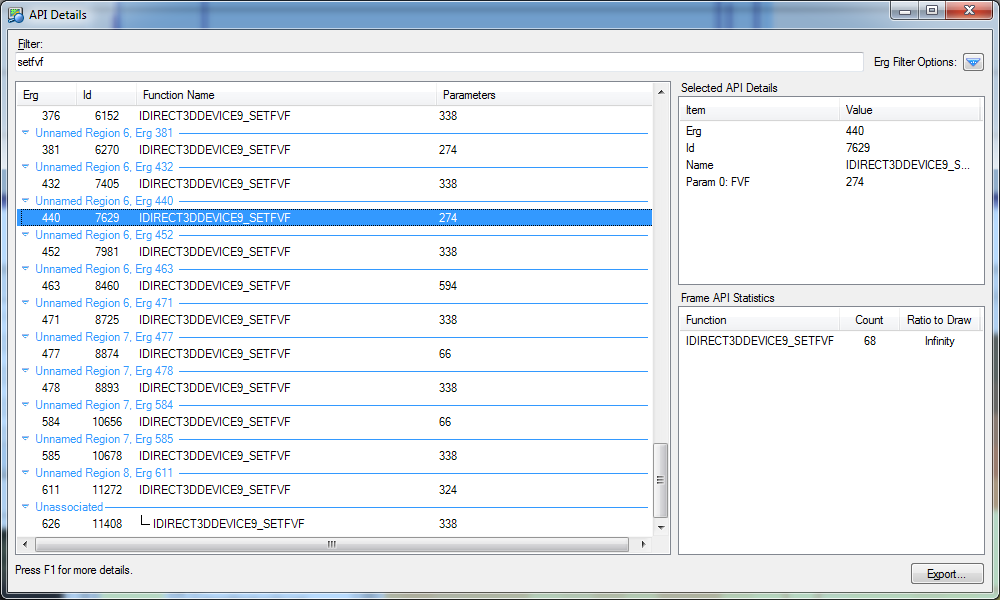Refactoring code from last time, I split into ply.py and ply2obj.py.

``````import struct

# constants
PLYMAGICK = "EPLYBNDS"
SUPPORTED_FORMAT = [0x0644]
class PLY:
def __init__(self, path):
self.path = path
self.indeces = []
self.positions = []
self.normals = []
self.UVs = []
self.open(self.path)

def open(self, peek=False, verbose=False):
with open(self.path, "rb") as f:
magick, = struct.unpack("8s", f.read(8))
if magick != PLYMAGICK:
raise Exception("Unsupported format")
x1, y1, z1, x2, y2, z2 = struct.unpack("ffffff", f.read(24))
mesh_entry, = struct.unpack("4s", f.read(4))
print "Mesh entry", mesh_entry
if mesh_entry != "MESH":
raise Exception("Unsupported type")
# read some unknown data
triangles, = struct.unpack("<I", f.read(4))
print "Number of triangles:",triangles
material_info, = struct.unpack("<I", f.read(4))
print "Material info", hex(material_info)
if material_info in SUPPORTED_FORMAT:
else:
raise Exception("Unsupported material type")
material_name_length, = struct.unpack("B", f.read(1))
print "Material file:", material_file
print "Vertices entry", verteces_entry
verts, = struct.unpack("<I", f.read(4))
print "Number of verts:", verts
vertex_description, = struct.unpack("<I", f.read(4))
print "Vertex description", hex(vertex_description)
for i in range(0, verts):
if vertex_description == 0x00010024:
vx,vy,vz,nx,ny,nz,U,V = struct.unpack("ffffff4xff", f.read(36))
elif vertex_description == 0x00070020:
vx,vy,vz,nx,ny,nz,U,V = struct.unpack("ffffffff", f.read(32))
else:
raise Exception("Unknown format: %s" % hex(vertex_description))
if verbose:
print "Vertex %i: " % i,vx,vy,vz
self.positions.append((vx,vy,vz))
self.normals.append((nx,ny,nz))
self.UVs.append((U,V))
print "Vertex info ends at:",hex(f.tell())
idx_count, = struct.unpack("<I", f.read(4))
#print "Indeces:", indeces
for i in range(0, idx_count/3):
i0,i1,i2 = struct.unpack("<HHH", f.read(6))
if verbose:
print "Face %i:" % i,i0,i1,i2
self.indeces.append((i0,i1,i2))
#print(hex(f.tell()))

def dump(self, outfile):
with open(outfile, "wb") as f:
for p in self.positions:
f.write('{:s} {:f} {:f} {:f}\n'.format("v", *p))
for UV in self.UVs:
u = UV
v = 1.0 - UV
f.write('{:s} {:f} {:f}\n'.format("vt", u, v))
for n in self.normals:
f.write('{:s} {:f} {:f} {:f}\n'.format("vn", *n))
for idx in self.indeces:
new_idx = map(lambda x: x+1, idx)
# change vertex index order by swapping the first and last indeces
f.write('{:s} {:d}/{:d}/{:d} {:d}/{:d}/{:d} {:d}/{:d}/{:d}\n'.format("f", new_idx, new_idx,
new_idx, new_idx, new_idx, new_idx, new_idx, new_idx, new_idx))``````

AK 47 has multiple MESH entries, a new material type highlighted in teal. I refactored the code to work as a state machine.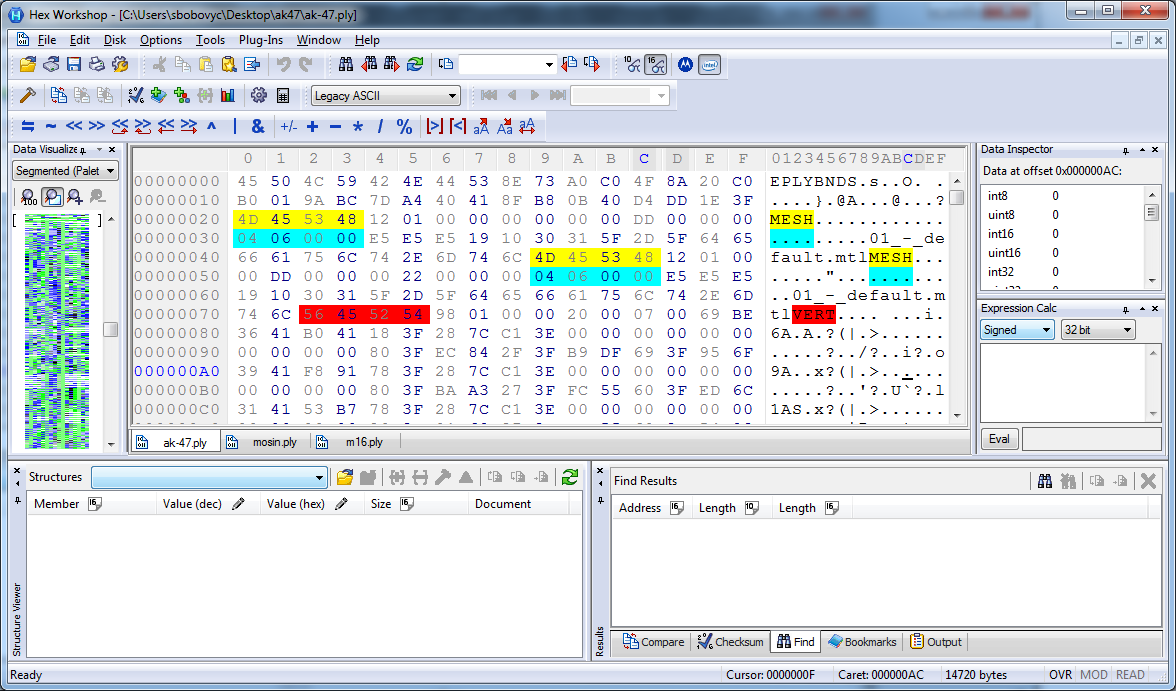``````import struct

# constants
PLYMAGICK = "EPLYBNDS"
SUPPORTED_ENTRY = ["MESH", "VERT", "INDX"]
SUPPORTED_FORMAT = [0x0644, 0x0604]

class PLY:
def __init__(self, path):
self.path = path
self.indeces = []
self.positions = []
self.normals = []
self.UVs = []
self.open(self.path)

def open(self, peek=False, verbose=False):
with open(self.path, "rb") as f:
magick, = struct.unpack("8s", f.read(8))
if magick != PLYMAGICK:
raise Exception("Unsupported format")
x1, y1, z1, x2, y2, z2 = struct.unpack("ffffff", f.read(24))
while True:
entry, = struct.unpack("4s", f.read(4))
print "Found entry", entry
if not(entry in SUPPORTED_ENTRY):
raise Exception("Unsupported entry type")
if entry == SUPPORTED_ENTRY: #MESH
# read some unknown data
triangles, = struct.unpack("<I", f.read(4))
print "Number of triangles:",triangles
material_info, = struct.unpack("<I", f.read(4))
print "Material info:", hex(material_info)
if material_info in SUPPORTED_FORMAT:
else:
raise Exception("Unsupported material type")
material_name_length, = struct.unpack("B", f.read(1))
print "Material file:", material_file
if entry == SUPPORTED_ENTRY: #VERT
verts, = struct.unpack("<I", f.read(4))
print "Number of verts: %i at %s" % (verts, hex(f.tell()))
vertex_description, = struct.unpack("<I", f.read(4))
print "Vertex description:", hex(vertex_description)
for i in range(0, verts):
if vertex_description == 0x00010024:
vx,vy,vz,nx,ny,nz,U,V = struct.unpack("ffffff4xff", f.read(36))
elif vertex_description == 0x00070020:
vx,vy,vz,nx,ny,nz,U,V = struct.unpack("ffffffff", f.read(32))
else:
raise Exception("Unknown format: %s" % hex(vertex_description))
if verbose:
print "Vertex %i: " % i,vx,vy,vz
self.positions.append((vx,vy,vz))
self.normals.append((nx,ny,nz))
self.UVs.append((U,V))
print "Vertex info ends at:",hex(f.tell())
if entry == SUPPORTED_ENTRY: #INDX
idx_count, = struct.unpack("<I", f.read(4))
print "Indeces:", idx_count
for i in range(0, idx_count/3):
i0,i1,i2 = struct.unpack("<HHH", f.read(6))
if verbose:
print "Face %i:" % i,i0,i1,i2
self.indeces.append((i0,i1,i2))
#print(hex(f.tell()))
break

def dump(self, outfile):
print "Dumping to OBJ"
with open(outfile, "wb") as f:
for p in self.positions:
f.write('{:s} {:f} {:f} {:f}\n'.format("v", *p))
for UV in self.UVs:
u = UV
v = 1.0 - UV
f.write('{:s} {:f} {:f}\n'.format("vt", u, v))
for n in self.normals:
f.write('{:s} {:f} {:f} {:f}\n'.format("vn", *n))
for idx in self.indeces:
new_idx = map(lambda x: x+1, idx)
# change vertex index order by swapping the first and last indeces
f.write('{:s} {:d}/{:d}/{:d} {:d}/{:d}/{:d} {:d}/{:d}/{:d}\n'.format("f", new_idx, new_idx,
new_idx, new_idx, new_idx, new_idx, new_idx, new_idx, new_idx))``````

MoW:Vietnam uses TGA files for textures and most models use both diffuse and specular textures.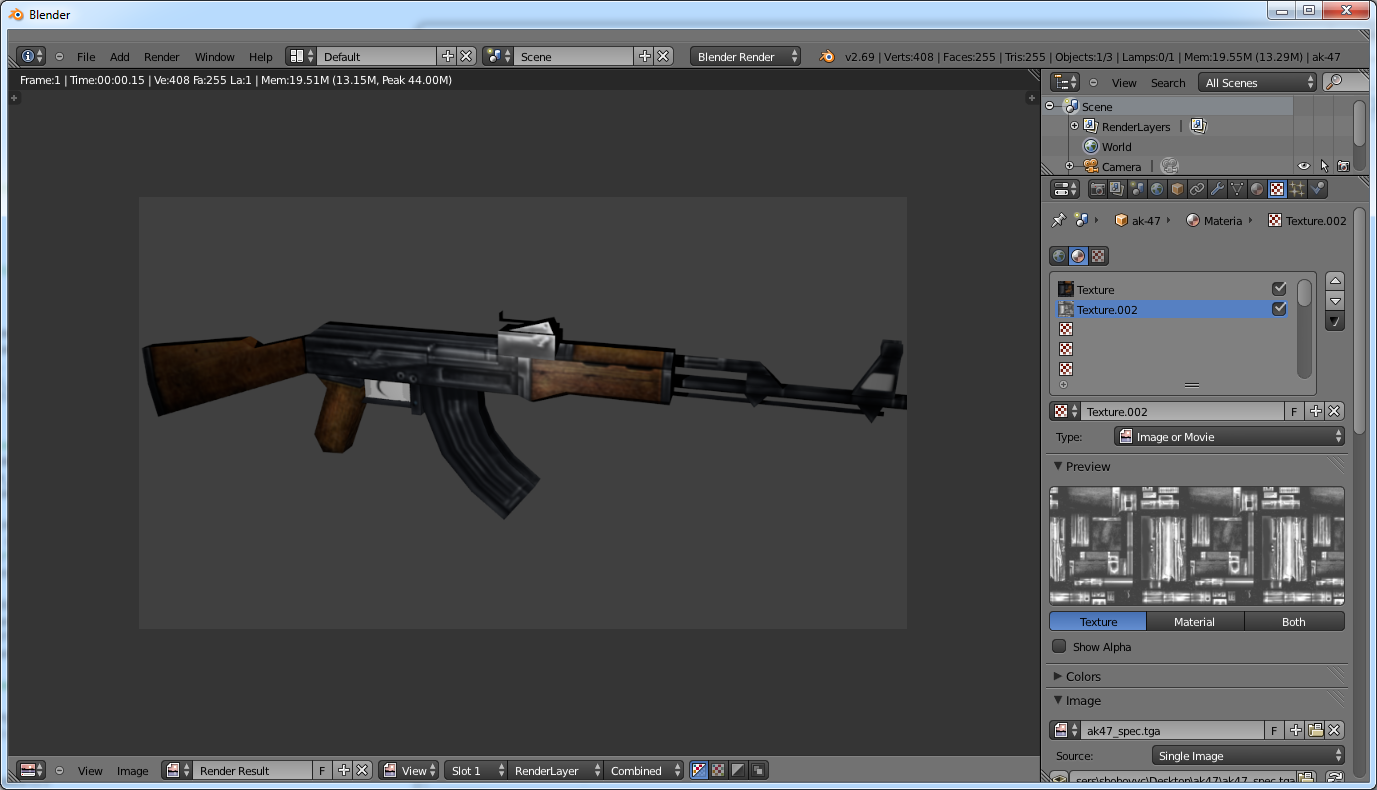Running the tool against m16.ply:

``````\$ python ply2obj.py m16/m16.ply
Found entry MESH
Number of triangles: 232
Material info: 0x404
Traceback (most recent call last):
File "ply2obj.py", line 18, in
p = ply.PLY(infile)
File "c:\Users\sbobovyc\Desktop\ply.py", line 15, in __init__
self.open(self.path)
File "c:\Users\sbobovyc\Desktop\ply.py", line 39, in open
raise Exception("Unsupported material type")
Exception: Unsupported material type``````

In addition, the 0x404 material immediately has the material file name following it instead of an unknown uint32. Here the triangle count is yellow, material type is is green, red is the length of the material file name.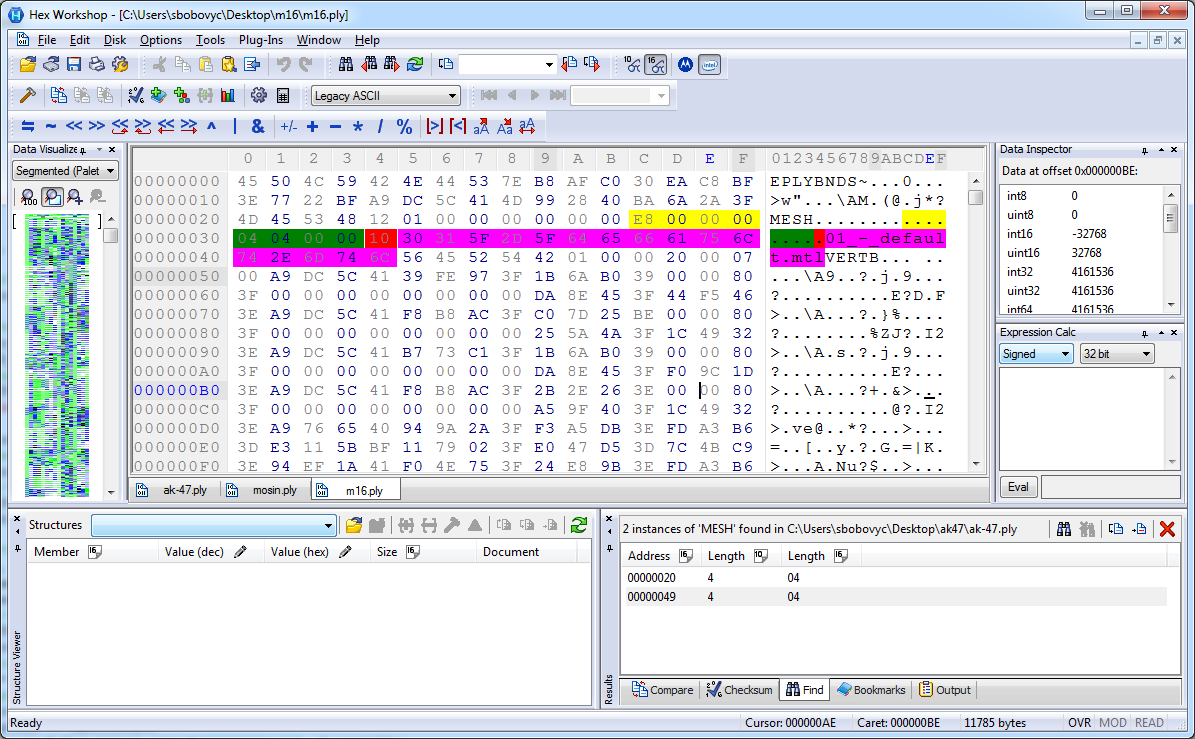A small modification fixes the problem:

``````if material_info in SUPPORTED_FORMAT:
if material_info == 0x0404:
pass
else:
else:
raise Exception("Unsupported material type")``````

Now, I wanted to tackle vehicle models. I used the tools against the chassis of the tank.

``````\$ python ply2obj.py t_54/body.ply
Found entry MESH
Number of triangles: 140
Material info: 0x404
0xa
Material file: engine.mtl
Found entry MESH
Number of triangles: 1658
Material info: 0x704
Traceback (most recent call last):
File "ply2obj.py", line 18, in
p = ply.PLY(infile)
File "c:\Users\sbobovyc\Desktop\ply.py", line 15, in __init__
self.open(self.path)
File "c:\Users\sbobovyc\Desktop\ply.py", line 42, in open
raise Exception("Unsupported material type")
Exception: Unsupported material type``````

After adding the material type to supported list:

``````\$ python ply2obj.py t_54/body.ply
Found entry MESH
Number of triangles: 140
Material info: 0x404
0xa
Material file: engine.mtl
Found entry MESH
Number of triangles: 1658
Material info: 0x704
0x8
Material file: mat1.mtl
Found entry VERT
Number of verts: 3970 at 0x68L
Vertex description: 0x70030
Traceback (most recent call last):
File "ply2obj.py", line 18, in
p = ply.PLY(infile)
File "c:\Users\sbobovyc\Desktop\ply.py", line 15, in __init__
self.open(self.path)
File "c:\Users\sbobovyc\Desktop\ply.py", line 58, in open
raise Exception("Unknown format: %s" % hex(vertex_description))
Exception: Unknown format: 0x70030``````

The length of vertex data for T54 body is 48 bytes long.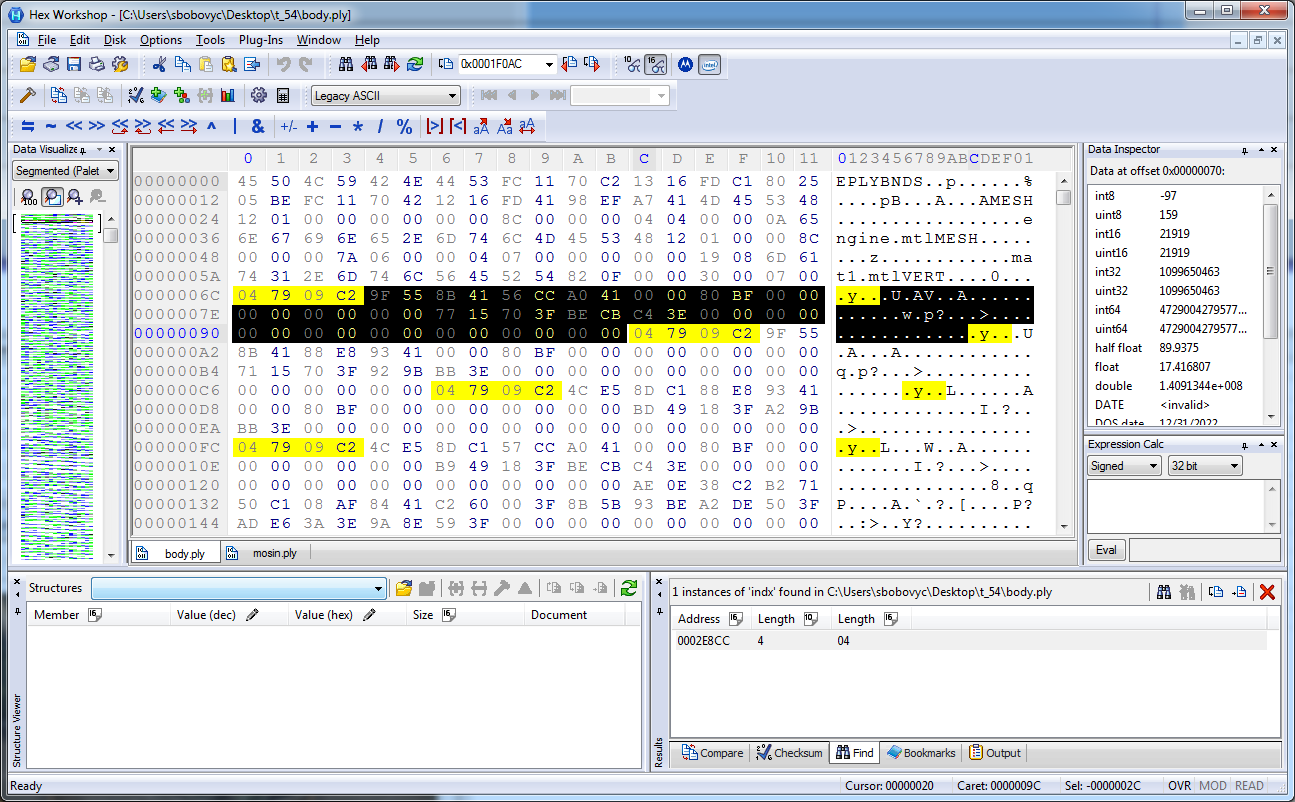Another slight modification to the code:

``````elif vertex_description == 0x00070030:
vx,vy,vz,nx,ny,nz,U,V = struct.unpack("ffffffff16x", f.read(48))``````

Here is the T54 chassis rendered in Blender: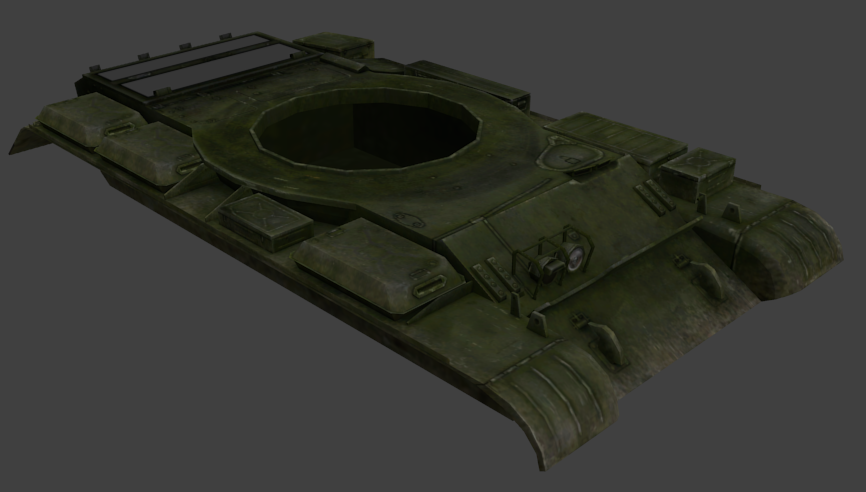# Conclusion

Reverse engineering of static models is progressing nicely. You can find the most current version of the code here https://github.com/sbobovyc/GameTools/tree/master/MoW